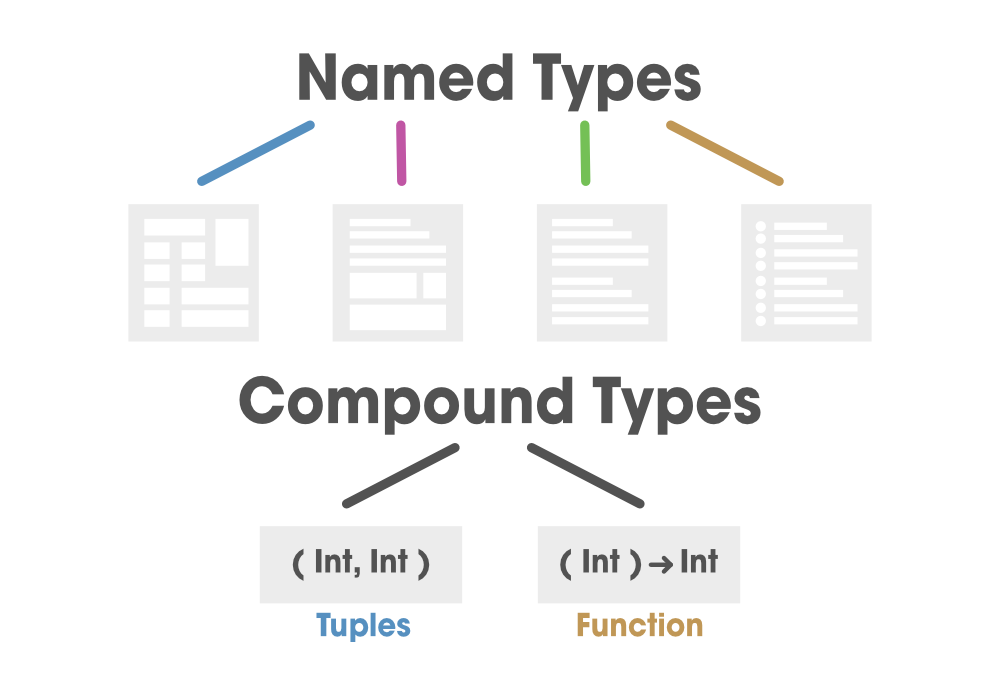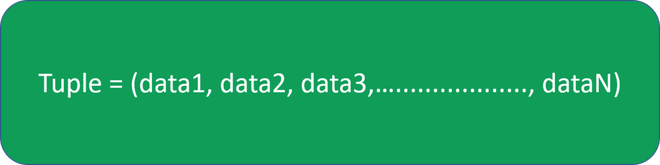GeeksforGeeks App
Open AppBrowser
Continue

A Tuple is a constant or variable that can accommodate a group of values that can be of different data types and compounded for a single value. In easy words, a tuple is a structure that can hold multiple values of distinct data types. Tuples are generally used as return values to retrieve various data from the output of any process.In order to create a tuple, first declare a tuple in Swift, we declare a constant or a variable in our code and type the data directly into the circular brackets separated with a comma. Below is the basic structure of a tuple where data1, data2, data3,……,dataN can all be of the same or distinct data type.Implementation:

Example 1: Declaring a tuple containing a string and an integer

```// statusLoad is of type (String, Int), and equals to (“Forbidden”, 403)

So, this data is available in two forms, String, a human-readable description, and Int, which is a number.

We can create a tuple from any permutation of data types, and that they can contain as many various types as you wish. Meaning, a tuple can hold any amount of data of similar or different data types.

Example 2: Declaring a tuple containing a string

```let a = (Int, Int, Int, Int)
var b = (String, Int, String, Int, Int)```

Additionally, we can name individual elements inside a tuple which is shown in the below example

Example 3:

`let statusLoad = (tupleMessage: “Forbidden”, tupleCode = 403)`

Now in order to decompose a tuple in swift, it is done and split into variables or constants . We can access the data in a tuple by decomposing it into constants and variables

It is as shown below in the example shown below:

Example 4:

```// refer statusLoad from above

// prints the message “Forbidden”
print(“The status message is \(sMessage).”)

// prints the code “403”
print(“The status code is \(sCode).”)```

Output:

```The status message is Forbidden.
The status code is 403.```

Note: Additionally, we can access a specific element inside a tuple by replacing the unwanted data positions with an underscore (_).

Example 5:

```let (sMessage,_) = statusLoad

print(“The status message is \(sMessage).”)
// prints the message “Forbidden”

print(“The status code is \(sCode).”)
// prints the code “403”```

Output:

```The status message is Forbidden.
The status code is 403.```

Note: Alternatively, we can access the data using indexed numbers. These numbers start from 0, meaning that we need to pass n-1 indexed term to access the nth element.

Example 6:

```let sMessage = statusLoad.0

// prints the message “Forbidden”
print(“The status message is \(sMessage).”)

// prints the code “403”
print(“The status code is \(sCode).”)```

Output:

```The status message is Forbidden.
The status code is 403.```

Refer to example 3 above illustrated:

Note: We can also access every individual element by their names as shown:

Example 7:

```let statusLoad = (tupleMessage: “Forbidden”, tupleCode = 403)

// prints the message “Forbidden”
```The status message is Forbidden.